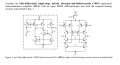# Finding the gain expression for this CMOS operational transconductance amplifier

#### asdasd12e12

Joined Nov 24, 2021
48
So here's the circuit:I think M2 is a common source amplifier and M4 a common gate amplifier, so they form a cascode amplifier where the gain is given by : Av = -gm2 * r0, r0 is the impedance at the node where the drain of the common gate amplifier is at (Vop).

r0 = ( [rds8 // (rds2a * ( gm4a / gds4a) )] * ( gm6 / gds6 ) ) // ( rds2 * (gm4 / gds4) )

I think the low frequency gain is this and there is no need to worry about the rest of the circuit because this circuit is symmetric.

So, is this how the gain is calculated or am i doing something wrong?

Also, i think transistors M5,M6,M7,M8 and the one where VDD is at are just for polarizing purposes but i dont know what's the purpose of transistors M1a,M2a,M3a and M4a. Why are they there?